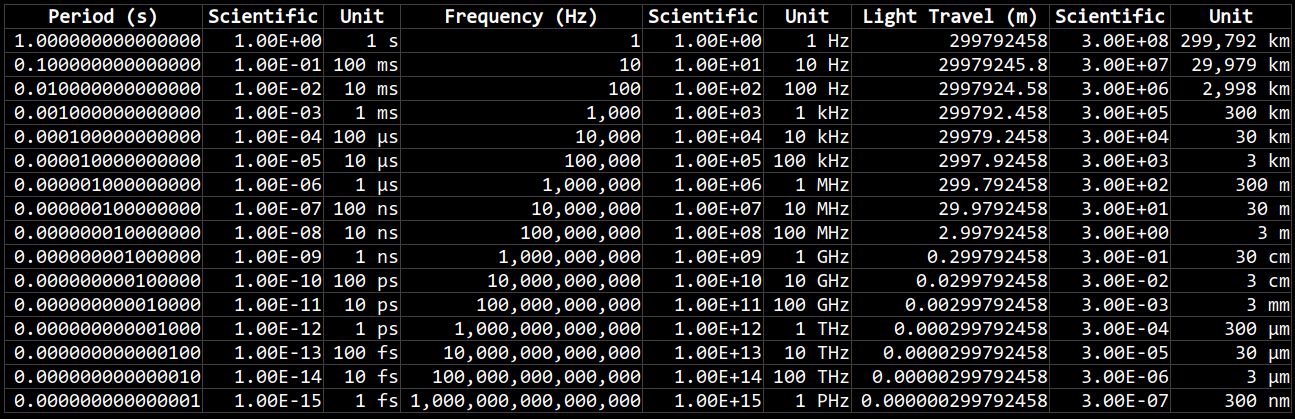# Period and Frequency

Oh, wait. I made it better.These charts show how period, frequency, and the speed of light are related to each other, in scientific and compact units.

Period is measured in seconds, frequency is measures in Hertz (cycles per second), and the distance of light travelled during one period is measured in meters (it is also known as the wavelength). The equation for wavelength is:

λ = c / ν

Where λ is the wavelength in meters, c is the speed of light in meters per second, and ν is the frequency of the wave in Hertz (cycles per second).

Wavelengths between 400 nanometers (nm) for violet light to 700 nm for red light are the visible component of the electromagnetic spectrum. The frequency of red light, on the low end of the visible spectrum, is about 430 terahertz (THz); whereas violet light, on the high end of the visible spectrum, is around 750 THz.